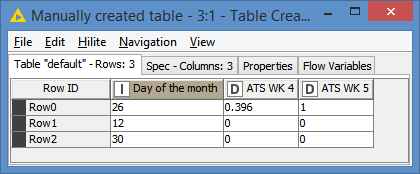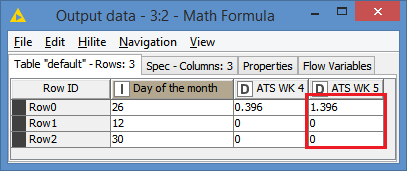# Knime rule engine based on date

Hi I would like to ask if i want to put …
If date of the month >23rd then data in ATS WK 4 column will be added to ATS WK 5.
If date of the month =< 23rd then ATS WK 4 data will remain.

my rule engine formula… but its wrong here
\$Day of month\$ > “23” = \$Sum(ATS WK4 MSU)\$ = \$Sum(ATS WK5 MSU)\$
TRUE => \$Day of month\$

Hi @Michellehml and welcome to KNIME Community Forum,

You can do this as a single step with Column Expressions or in 2 steps with Math Formula and Rule Engine.

Here is an example:
40528.knwf (6.5 KB)

2 Likes

Hi Armingrud, thank you.

I do not have column expression node (4.4.2 knime) and i cannot seem to download the extension also. Therefore I will be using math formula and rule engine.

Can you screen shot and upload the example as an image? because I cannot access the example you provided.

Thank you in advancedFor KNIME 4.4.x you can download the “KNIME Expressions” extension from this update site:
https://update.knime.org/analytics-platform/4.4
Then you can use the “Column Expressions” node.

To do the same thing using KNIME base nodes, you can calculate the sum of wk4 and wk5 in Math Formula then use Rule Engine to replace the value of wk5 if “day of month” is greater than 23. Something like this:

``````\$day of month\$ > 23 => \$sum of wk4 and wk5\$
TRUE => \$wk5\$
``````

And replace wk5 in the node configurations.
You can use the same condition to change the value of wk4 to zero in another Rule Engine node.

1 Like

alright thank you.

This one ^ the output is correct but i notice on 5th of the month, knime will take wk4+5 too

Can I know where the error in this formula? I want if less than or equals to 23rd of the month, the wk 4 will become 0 because it is ady added to week 5

Day of month should be a numeric value not nominal. As a nominal (String) value, “5” is greater than “23”. Like when you sort them in ascending order and “5” comes after “23”.

1 Like

Hi Armingrud, my day of the month column is in integer. I change the column to decimal as well. Same issue.

The issue only occurs on the 5th day of the month and im unsure why because the 19th day works fine…

That’s because you are using a string value in your condition: “23”. Use this instead:
`\$day of month\$ > 23`
Remove double quotations around 23. You don’t need to convert “day of month” to double, integer is fine.

2 Likes

Hi @Michellehml , besides what @armingrudd has explained, it looks to me that you might not understand the syntax of the Rule Engine.

The basic syntax is:

``````Condition(s) => value
TRUE => default value
``````

which translate to:
if `Condition(s)` then `value`
else (if `Condition(s)` did not satisfy) `default value`

So, your initial expression is invalid:
`\$Day of month\$ > “23” = \$Sum(ATS WK4 MSU)\$ = \$Sum(ATS WK5 MSU)\$`

Similarly, your second attempt is also invalid:
`\$Day of month\$ > “23” => \$Sum(ATS WK4 MSU)\$ = "0"`

You can’t have `\$Sum(ATS WK4 MSU)\$ = "0"` as value. You can’t have an equation as value, that is why you are getting the error.

Then this should become an additional rule. You rules should be:

``````\$Day of month\$ > 23 => \$Sum(ATS WK4 MSU)\$
\$Day of month\$ <= 23 => 0
TRUE => \$Sum(ATS WK4 MSU)\$
``````

Now, since the conditions `\$Day of month\$ > 23` and `\$Day of month\$ <= 23` are the only possible conditions, this means that if you are not in one, you are in the other, therefore you can just evaluate one of the condition and set the default value for the other condition. This can be written like this:

``````\$Day of month\$ > 23 => \$Sum(ATS WK4 MSU)\$
TRUE => 0
``````

EDIT: I am assuming that the column name is `Sum(ATS WK4 MSU)`. Columns are referenced by `\$column name\$`. If you meant to do a sum, you cannot do this in the Rule Engine. You either have to pre-compile the sum in a column, which you can assign as value, or you can use the Column Expression node instead which allows you to do operations as `value`

1 Like

Hi @Michellehml , I went back to your first post to understand what you are trying to do (and it does look like you are trying to do a sum). And welcome to the Knime Community.

You can do this in the Math Formula, since you are dealing with numbers, and the Math Formula does offer the ability to use if condition. However, like almost all nodes, the result of one Math Formula node will be for one column, and since you are modifying 2 columns (your initial rules are incomplete: see the clarified version below), you will need 2 Math Formula. The Column Expression node would allow you to do both in the same node, however, you would still need to duplicate the same rules.

I’ll do both versions for you. Before this, however, I want to clarify the rules:

``````If Day of the month > 23:
ATS WK 5 = ATS WK4 + ATS WK5
ATS WK 4 = 0 (this is not specified, but is demonstrated in your output)
else (that is if Day of the month <= 23):
No change in either column
``````

The workflow with both methods looks like this:Input data (same as yours):Let’s look at the Math Formula method:
I first process the ATS WK 5, since I need the original value of ATS WK 4 (If I process ATS WK 4 first, I will lose its original value since processing ATS WK 4 means setting it to 0).

The Expression for this is:
`if(\$Day of the month\$ > 23, \$ATS WK 4\$ + \$ATS WK 5\$, \$ATS WK 5\$)`

This means if `\$Day of the month\$ > 23`, set value to `\$ATS WK 4\$ + \$ATS WK 5\$`, otherwise keep the same value of `\$ATS WK 5\$` (don’t forget that we are processing the column \$ATS WK 5\$ here)

So, at this point, `ATS WK 5` has been processed and we can see the resultsNow we process `ATS WK 4`, so onto the next Math Formula:
`if(\$Day of the month\$ > 23, 0, \$ATS WK 4\$)`

Similarly, this is saying if `\$Day of the month\$ > 23`, set value to 0, otherwise keep `\$ATS WK 4\$` as is.

Final results:Let’s look at the Column Expression now:

As you can see, I have 2 expressions defined. The screenshot is showing the process for ATS WK 5:

``````if(column("Day of the month") > 23) {
column("ATS WK 4") + column("ATS WK 5")
} else {
column("ATS WK 5")
}
``````

This translate to the same thing as what we did in the Math Formula. If `Day of the month > 23` then set value to `ATS WK 4 + ATS WK 5`, otherwise leave as `ATS WK 5`

And I’m making sure that the respective expression is replacing its respective column:

And here’s the expression for `ATS WK 4`:

``````if(column("Day of the month") > 23) {
0
} else {
column("ATS WK 4")
}
``````

Final result:Here’s the workflow: math formula with conditions.knwf (9.5 KB)

Note: I know you mentioned that you don’t have the Column Expression and you are struggling to download it. By opening my workflow, Knime should offer you to automatically download and install the proper version of Column Expression for your Knime version.

1 Like

Hi thank you very much @armingrudd and @bruno29a for the very detailed explaination. I genuinely appreciate it a lot especially im super new to knime and data analysis…

I got it figured out already and i use 1 math formula node and 2 rule engines because i dont have column expression node yet.

Thank you very much and have a great day aheadThis topic was automatically closed 90 days after the last reply. New replies are no longer allowed.